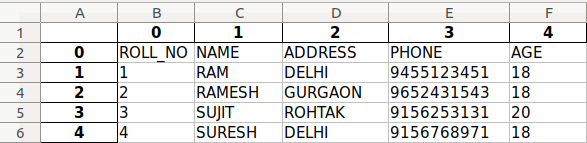# Python | Convert an HTML table into excel

• Difficulty Level : Basic
• Last Updated : 25 Jun, 2019

MS Excel is a powerful tool for handling huge amounts of tabular data. It can be particularly useful for sorting, analyzing, performing complex calculations and visualizing data. In this article, we will discuss how to extract a table from a webpage and store it in Excel format.

Step #1: Converting to Pandas dataframe
Pandas is a Python library used for managing tables. Our first step would be to store the table from the webpage into a Pandas dataframe. The function `read_html()` returns a list of dataframes, each element representing a table in the webpage. Here we are assuming that the webpage contains a single table.

 `# Importing pandas``import` `pandas as pd`` ` `# The webpage URL whose table we want to extract``url ``=` `"https://www.geeksforgeeks.org/extended-operators-in-relational-algebra/"`` ` `# Assign the table data to a Pandas dataframe``table ``=` `pd.read_html(url)[``0``]`` ` `# Print the dataframe``print``(table)`

Output

```         0       1        2           3    4
0  ROLL_NO    NAME  ADDRESS       PHONE  AGE
1        1     RAM    DELHI  9455123451   18
2        2  RAMESH  GURGAON  9652431543   18
3        3   SUJIT   ROHTAK  9156253131   20
4        4  SURESH    DELHI  9156768971   18
```

Step #2: Storing the Pandas dataframe in an excel file
For this, we use the to_excel() function of Pandas, passing the filename as a parameter.

 `# Importing pandas``import` `pandas as pd`` ` `# The webpage URL whose table we want to extract``url ``=` `"https://www.geeksforgeeks.org/extended-operators-in-relational-algebra/"`` ` `# Assign the table data to a Pandas dataframe``table ``=` `pd.read_html(url)[``0``]`` ` `# Store the dataframe in Excel file``table.to_excel(``"data.xlsx"``)`

Output:In case of multiple tables on the webpage, we can change the index number from 0 to that of the required table.

My Personal Notes arrow_drop_up